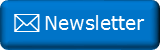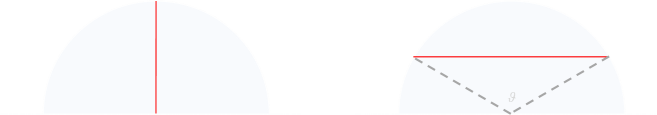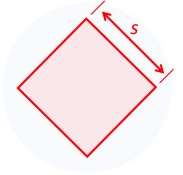﻿ Cake cuttingNewsletter: Click here to join an email list and be contacted when a new article is published.      Advertisement

# Cake cutting

Today I'm going to look at an alternate (more efficient?) way to cut a cake into eight equal sized pieces using straight cuts. Notice that I said equal sized pieces. This does not mean they have to be equal shaped pieces.We can examine this problem in a recursive way. First cutting the cake in half, then these pieces in half, then these pieces in half again.

Let’s say you are the owner of a delicious round section (cylindrical) cake, and you want to bisect this cake into two equal sized pieces. You want to do this using a single straight cut. What is the shortest length cut you make to do this?(Throughout this post we’re going to add the restriction that we’ll be making all cuts perpendicular to the round face of the cake; treating it like a circle. Of course it is possible to bisect a cake by slicing it through the middle, but that's too easy. Also, if the cake happened to have icing on the top, then cutting through the middle would result in one piece having all the icing, and one none. That's not fair!)

We're also going to add the restriction that we're not allowed to move pieces between cuts.

## Cut it in halfThere’s really only way to this: a straight line through the center of the cake. The length of this cut will be the diameter of the cake. If we normalize our cake to say it has a radius of one unit, then the cut is two units in length.
 Out of interest, if we elected to bisect the cake with a circular cut we’d need to cut the cake to a new radius of 1/√2 of the original radius. This would create a new circular cake with half the volume, and an annulus cake (donut shape) with the other half. The circumerfance of this cut would be π√2, which is over twice the length of the bisecting diameter cut. Fancy, but not efficient, and messy to cut.## Cut it in quarters

Things get a little more interesting if we want to cut the cake in half again. Starting with the semi-circular slab of cake, if we’re using a straight cut, we have a range of options between two extremes. At one end of the spectrum we can slice it in half using a cut along the radius (shown left below). At the other end of the spectrum we can lop off a segment from the edge (shown below right), using a cut direction perpendicular to the radius cut. There are an infinite number of solutions inbetween with the cut being at an arbitrary angle.It’s pretty clear the radius cut is the shortest, and with our normalized cake the cut will be of length one unit.

If we did try and cut off half the cake as a segement, how long a cut would that be?

The formula for the area of the top segment is given below (where θ is measured in radians):It’s a pretty easy formula to understand, it’s simply the area of the pie-shaped sector of the circle, subtract the area of the triangle piece.

With the angle determined, the length of the chord can be calculated.

To carve away half the cake, using the formula above, we can determine that the angle is θ ≈ 2.31rad (≈132°)

Using trigonometry, the length of the straight cut is 2rsin(θ/2) ≈ 1.83 units.

The distance this is away from the base of the semi-circle is rcos(θ/2) ≈ 0.404 units.Interestingly, these are well-used formulas, as they have applications for things such as determining the volume of fluid in a circular tank laid on its side. They can also be used to determine the shape of a circular segmented transom window over a doorway (if an architect knows the width of the top of the doorway, and the height she wants to make the window, then the related formula below can be used to determine the radius of the circle that meets these two criteria).

The height of the segment is given a fancy name of sagitta, and if you read my previous article on Vestigial Trig Functions you might appreciate that the sagitta is the Versin of half the angle of the sector.As the redius cut is length one unit, and the segment cut is 1.83 units, it's more efficient to cut into quarters along the radius.

## Cut it in eighths

Now things get really interesting. With the two most efficient straight cuts we've got ourselves a quarter piece of cake. What is the most efficient way to bisect this quarter piece with the next straight cut?

There are three ways we might consider cutting: Radius cut, vertical cut (or horizontal with rotational symmetery), or a diagonal cut.The radius cut, as before, is one unit long. I've drawn it at 45° in the diagram above left, but since it's the radius I could just as easily have drawn it vertically. From this thought experiment, we can see that the middle cut (the vertical cut) has to be shorter than this.

If we cut the cake with the vertical cut (middle above), from symmetry, we can see that the length of this vertical cut is half the length of the segment cut in the semicircle exercise above, giving it a length of ≈ 0.915 units. This cut will be ≈ 0.404 units from the edge.

We can confirm this with a little calculus. The equation for the circle is below, and with normalizing the radius to one and rearranging we can get a formula for y with respect to x.We can integrate this from the axis of x=0, to a position x=h along so that the area under the curve is an eighth of the area of a circle (in our case π/8). A little bit of calculus later shows that this value for h corresponds to the value we determined early of the distance of the chord from the axis.For the diagonal case we can imagine four corner pieces joined back together. The square with side S will have an area of half of that of the circle. The area of the normalized cake is π units2, so the area of the square will be π/2 units2. This means the length of each side of the square, and thus the length of the cut is √(π/2) ≈ 1.253 units. The vertices of the square cross the x and y axes at (√π)/2 ≈ 0.886 units.

The vertical cut is the most efficient way to cut a quarter piece of cake into two equal pieces.

## Putting it all together

It's possible to combine our results.

Putting all this work together, next time you are asked to cut a cake into eight equal pieces, which way will you do it?Even though the radial way makes pieces of equal shape (shown left), the method on the right not only results in less cutting, but, depending on the texture of the cake, might end up with neater pieces. Using a traditional sector cut, towards the center where the pieces get narrow, it's easy for the knife to crush and distort a delicate cake, and hard to make a defined edge. This is not as much of a problem when the cuts are perpendicular and spaced further apart.

## Update

If you remove the restriction that cuts have to go from edge to edge you can reduce the total length of the cuts even further.

You can find a complete list of all the articles here.Click here to receive email alerts on new articles.